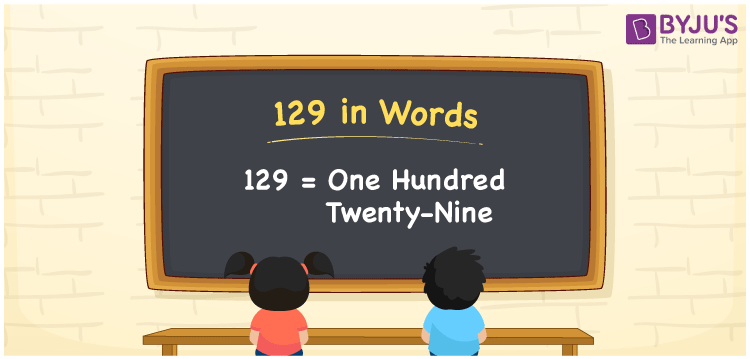# 129 in words

129 in words is written as One Hundred and Twenty Nine. 129 represents the count or value. The article on Counting Numbers can give you an idea about count or counting. The number 129 is a 3 digit number that is used in expressions related to money, days, distance, length, weight and so on. Let us consider an example for 129. “I have One Hundred and Twenty Nine unique coins.”

 129 in words One Hundred and Twenty Nine One Hundred and Twenty Nine in Numbers 129

## 129 in English Words## How to Write 129 in Words?

We can convert 129 to words using a place value chart. The number 129 has 3 digits, so let’s make a chart that shows the place value up to 3 digits.

 Hundreds Tens Ones 1 2 9

Thus, we can write the expanded form as:

1 × Hundred + 2 × Ten + 9 × One

= 1 × 100 + 2 × 10 + 9 × 1

= 129

= One Hundred and Twenty Nine.

129 is the natural number that is succeeded by 128 and preceded by 130.

129 in words – One Hundred and Twenty Nine.

Is 129 an odd number? – Yes.

Is 129 an even number? – No.

Is 129 a perfect square number? – No.

Is 129 a perfect cube number? – No.

Is 129 a prime number? – No.

Is 129 a composite number? – Yes.

## Solved Example

1. Write the number 129 in expanded form

Solution: 1 × 100 + 2 × 10 + 9 × 1

We can write 129 = 100 + 20 + 9

= 1 × 100 + 2 × 10 + 9 × 1.

## Frequently Asked Questions on 129 in words

Q1

### How to write the number 129 in words?

129 in words is written as One Hundred and Twenty Nine.
Q2

### Is 129 divisible by 3?

Yes. 129 is divisible by 3.
Q3

### Is 129 a prime number?

No. 129 is not a prime number.